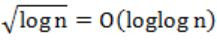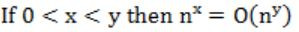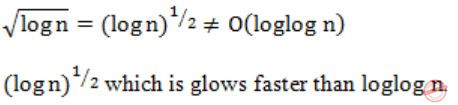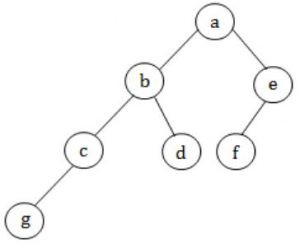## GATE 1996

 Question 1

Let A and B be sets and let Ac and Bc denote the complements of the sets A and B. The set (A – B) ∪ (B - A) ∪ (A∩B) is equal to

 A A ∪ B B Ac ∪ Bc C A ∩ B D Ac ∩ Bc
Question 1 Explanation:
(A – B) ∪ (B - A) ∪ (A∩B)(A - B) = 1
(B - A) = 2
(A∩B) = 3
A∪B = (1∪2∪3)
(A – B) ∪ (B - A) ∪ (A∩B) = 1∪2∪3 = (A∪B)
 Question 2

Let X = {2,3,6,12,24}, Let ≤ be the partial order defined by X ≤ Y if x divides y. Number of edge as in the Hasse diagram of (X,≤) is

 A 3 B 4 C 9 D None of the above
Question 2 Explanation:No. of edges = 4
 Question 3

Suppose X and Y are sets and X Y and are their respective cardinalities. It is given that there are exactly 97 functions from X to Y. from this one can conclude that

 A |X| = 1, |Y| = 97 B |X| = 97, |Y| = 1 C |X| = 97, |Y| = 97 D None of the above
Question 3 Explanation:
From the given information we can write,
|Y||X| = 97
→ Option A only satisfies.
 Question 4

Which of the following statements is false?

 A The set of rational numbers is an abelian group under addition. B The set of integers in an abelian group under addition. C The set of rational numbers form an abelian group under multiplication. D The set of real numbers excluding zero in an abelian group under multiplication.
Question 4 Explanation:
Rational number consists of number '0'. If 0 is present in a set inverse is not possible under multiplication.
 Question 5

Two dice are thrown simultaneously. The probability that at least one of them will have 6 facing up is

 A 1/36 B 1/3 C 25/36 D 11/36
Question 5 Explanation:
1 - no. 6 on both dice
1 - (5/6 × 5/6) = 1 - (25/36) = 11/36
 Question 6

The formula used to compute an approximation for the second derivative of a function f at a point X0 is

 A f(x0+h) + f(x0-h)/2 B f(x0+h) - f(x0-h)/2h C f(x0+h) + 2f(x0) + f(x0-h)/h2 D f(x0+h) - 2f(x0) + f(x0-h)/h2
Question 6 Explanation:
The formula which is used to compute the second derivation of a function f at point X is
f(x0+h) - 2f(x0) + f(x0-h)/h2
 Question 7

Let Ax = b be a system of linear equations where A is an m × n matrix and b is a m × 1 column vector and X is a n × 1 column vector of unknowns. Which of the following is false?

 A The system has a solution if and only if, both A and the augmented matrix [A b] have the same rank. B If m < n and b is the zero vector, then the system has infinitely many solutions. C If m = n and b is non-zero vector, then the system has a unique solution. D The system will have only a trivial solution when m = n, b is the zero vector and rank (A) = n.
Question 7 Explanation:
→ It belongs to linear non-homogeneous equations. So by having m=n, we can't say that it will have unique solution.
→ Solution can be depends on rank of matrix A and matrix [A B].
→ If rank[A] = rank[A B] then it can have solution otherwise no solution.
 Question 8

Which two of the following four regular expressions are equivalent? (ε is the empty string).

(i) (00)*(ε+0)
(ii) (00)*
(iii) 0*
(iv) 0(00)*
 A (i) and (ii) B (ii) and (iii) C (i) and (iii) D (iii) and (iv)
Question 8 Explanation:
(00)*(ε+0),0*
In these two, we have any no. of 0's as well as null.
 Question 9

Which of the following statements is false?

 A The Halting problem of Turing machines is undecidable. B Determining whether a context-free grammar is ambiguous is undecidbale. C Given two arbitrary context-free grammars G1 and G2 it is undecidable whether L(G1) = L(G2). D Given two regular grammars G1 and G2 it is undecidable whether L(G1) = L(G2).
Question 9 Explanation:
Equivalence of regular languages is decidable under
1) Membership
2) Emtiness
3) Finiteness
4) Equivalence
5) Ambiguity
6) Regularity
7) Everything
8) Disjointness
All are decidable for Regular languages.
→ First 3 for CFL.
→ Only 1st for CSL and REC.
→ None for RE.
 Question 10

Let L ⊆ Σ* where Σ = {a, b}. Which of the following is true?

 A L = {x|x has an equal number of a's and b's } is regular B L = {anbn|n≥1} is regular C L = {x|x has more a's and b's} is regular D L = {ambn|m ≥ 1, n ≥ 1} is regular
Question 10 Explanation:
L = {ambn|m ≥ 1, n ≥ 1}
Here, m and n are independent.
So 'L' Is Regular.
 Question 11

Which of the following is false?

 ABCDQuestion 11 Explanation:Question 12

Consider the following statements:

(i) First-in-first out types of computations are efficiently supported by STACKS.
(ii) Implementing LISTS on linked lists is more efficient than implementing LISTS on an array for almost all the basic LIST operations.
(iii) Implementing QUEUES on a circular array is more efficient than implementing QUEUES on a linear array with two indices.
(iv) Last-in-first-out type of computations are efficiently supported by QUEUES.

Which of the following is correct?

 A (ii) and (iii) are true B (i) and (ii) are true C (iii) and (iv) are true D (ii) and (iv) are true
Question 12 Explanation:
(i) FIFO computation efficiently supported by queues.
(iv) LIFO computation efficiently supported by stacks.
Then given (i) and (iv) are false.
 Question 13

An advantage of chained hash table (external hashing) over the open addressing scheme is

 A Worst case complexity of search operations is less? B Space used is less C Deletion is easier D None of the above
Question 13 Explanation:
In chained hash tables have advantages over open addressed hash tables in that the removal operation is simple and resizing can be postponed for longer time.
 Question 14

In the balanced binary tree in the below figure, how many nodes will become unbalanced when a node is inserted as a child of the node “g”?A 1 B 3 C 7 D 8
Question 14 Explanation:a, b, c are going to unbalance.
 Question 15

Which of the following sequences denotes the post order traversal sequence of the tree of question 14?

 A f e g c d b a B g c b d a f e C g c d b f e a D f e d g c b a
Question 15 Explanation:
Postorder:-
Left → Right → Root
g c d b f e a
 Question 16

Relative mode of addressing is most relevant to writing

 A coroutines B position – independent code C shareable code D interrupt handlers
Question 16 Explanation:
The main advantage of PC- relative addressing is that code may be position independent, i.e., it can be loaded anywhere in memory without the need to adjust any address.
 Question 17

The pass number for each of the following activities

1. Object code generation
2. Literals added to literal table
3. Listing printed
4. Address resolution of local symbols

That occur in a two pass assembler respectively are

 A 1, 2, 1, 2 B 2, 1, 2, 1 C 2, 1, 1, 2 D 1, 2, 2, 2
Question 17 Explanation:
The functionalities from pass 1 and pass 2 are:
Pass 1:
1) Assign addresses to all statements in the program.
2) Save the values assigned to all labels for use in pass 2.
3) Perform some processing of assembler directives.
Pass 2:
1) Assemble instructions.
2) Generate data values defined by BYTE, WORD etc.
3) Perform processing of assembler directives not done during pass 1.
4) Write the program and assembling listing.
 Question 18

The process state transition diagram in below figure is representative ofA a batch operating system B an operating system with a preemptive scheduler C an operating system with a non-preemptive scheduler D a uni-programmed operating system
Question 18 Explanation:
Transaction from running → ready present.
So this is preemptive.
There are 18 questions to complete.

Register Now